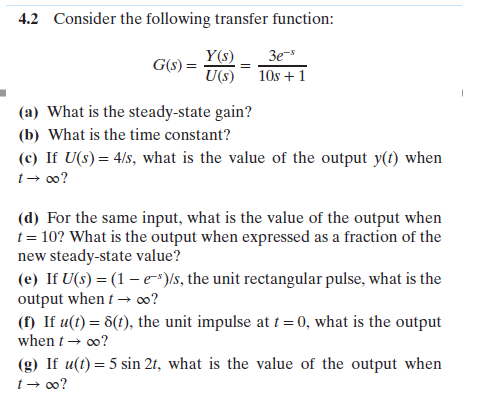# 4.2Consider the following transfer function:Y(s) 3e-U(s) 1Os +1(a) What is the steady-state gain?(b) What is the time constant?(c) If U(s)4/s, what is the value of the output y(t) whent oo?(d) For the same input, what is the value of the output whent 10? What is the output when expressed as a fraction of thenew steady-state value?(e) If U(s) (1-e-)s, the unit rectangular pulse, what is theoutput whent-oo?( If u() 6(), the unit impulse at 0, what is the outputwhen-00?(g) If u(t) 5 sin 2t, what is the value of the output when

Questionhelp_outlineImage Transcriptionclose4.2 Consider the following transfer function: Y(s) 3e- U(s) 1Os +1 (a) What is the steady-state gain? (b) What is the time constant? (c) If U(s)4/s, what is the value of the output y(t) when t oo? (d) For the same input, what is the value of the output when t 10? What is the output when expressed as a fraction of the new steady-state value? (e) If U(s) (1-e-)s, the unit rectangular pulse, what is the output whent-oo? ( If u() 6(), the unit impulse at 0, what is the output when-00? (g) If u(t) 5 sin 2t, what is the value of the output when fullscreen
check_circleExpert Solution
Step 1

Note: Since we only answer up to 3 sub-parts, we’ll answer the first 3. Please resubmit the question and specify the other subparts (up to 3) you’d like answered.

Part (a)

The general form of the transfer function for the given problem is written below.

Here, Kp is the steady-state gain.

τ is the time constant.

e-st is the transfer function for the transportation lag.

Step 2

Compare the given transfer function with the given transfer function to get the value of Kp (steady-state gain).

Step 3

Part (b)

Compare the given transfer function with the given transf...

### Want to see the full answer?

See Solution

#### Want to see this answer and more?

Solutions are written by subject experts who are available 24/7. Questions are typically answered within 1 hour*

See Solution
*Response times may vary by subject and question
Tagged in

### Chemical Engineering